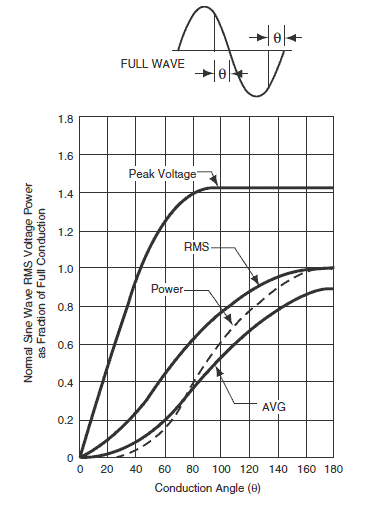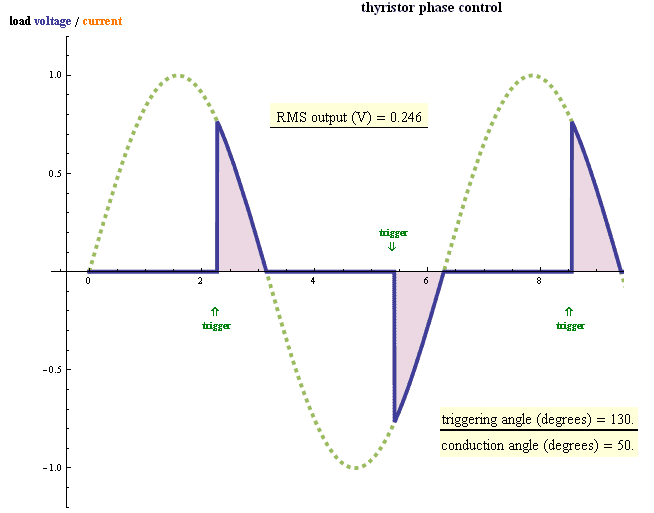# How to calculate RMS voltage from triac phase angle

Hi,
In a system that uses a triac to switch mains voltage, how can one calculate the resultant peak voltage, RMS voltage and power for a given phase (conduction) angle e.g. 50°?

Many thanks

in the usual way. Draw the output wave form graphs. That will directly give you peak.
RMS volatge = Sqrt of avg of square of instantaneous voltage. This you calculate by integration. And for finding the power, you need wave-form of current as well.
The you find power = Avg of ( V(instantaneous) * I(instantaneous) ) over a cycle.
In short, you need to do some work. :)
And, welcome of PF.

Drawing graphs is one way but I need a more technical solution. I need the formula!

Just a mathematical way to calculate the peak voltage based on a phase angle would be sufficient.
Here's hoping...

DC12, I believe if you draw some graphs of output voltage wave-forms for few values of firing angle (like, 30, 90, 150, 300 etc), then the formula you are trying to find will be apparent.
Sorry, but we can't do your homework here.

Thanks again I_am_learning. Graphs don't help at all. This needs to be done many times for many different levels and numerous angles - I just don't have enough ink and paper. No homework being done here sir.

Thanks again I_am_learning. Graphs don't help at all. This needs to be done many times for many different levels and numerous angles - I just don't have enough ink and paper. No homework being done here sir.

I think he's saying just work out one or a few cases, and find the relationships. Then you can apply what you see to a general case. I would tell you, but I don't know an equation for this off the top of my head.

I think that equation for RMS voltage across the load look like this:

$$V_{load} = Vpeak *\sqrt{\frac{2 \pi - 2 \varphi + sin2 \varphi}{4\pi}}$$

Where $$\varphi - triac- angle- delay = triggering -angle$$ .

$$for-0\varphi - triac- is- full- on$$ .

$$And for- \frac{\pi}{2} V_{load} = Vpeak/2$$ .

Or use this graphLast edited:
I thought that I shows some example.
For V = 230V we have 325V peak and conduction angle 50° so the triggering angle must be equal to 130°.First we must convert 130° degrees to radians:

r = 130 * ∏/180 = 130 * 0.0174 = 2.26 [rad]

I use Wolframalpha
http://www.wolframalpha.com/input/?i=sqr(+(2pi+-+2*2.26+++sin(2*2.26))/(4pi)+)

but you could use Google too

Code:
sqr( (2pi - 2*2.26 + sin(2*2.26))/(4pi) )

Vload = 325V * √ ( (2*pi - 2*r + sin(2r) ) / (4pi) ) = 325V * 0.249 = 80.9Vrms

Last edited:
sophiecentaur